Скачать презентацию INTERNATIONAL FINANCIAL MANAGEMENT Fifth Edition EUN RESNICK

• Количество слайдов: 61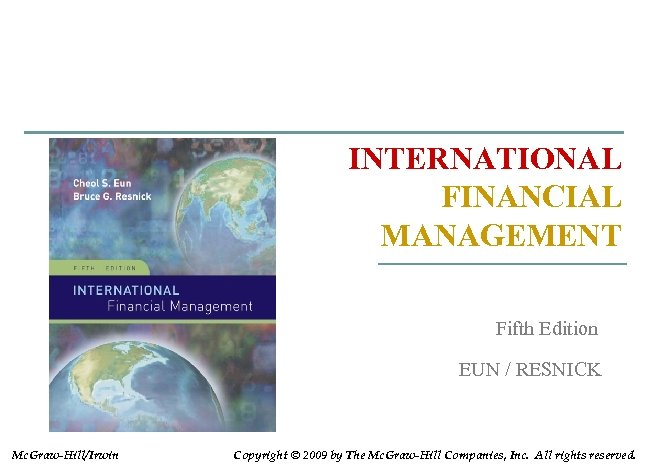INTERNATIONAL FINANCIAL MANAGEMENT Fifth Edition EUN / RESNICK Mc. Graw-Hill/Irwin Copyright © 2009 by The Mc. Graw-Hill Companies, Inc. All rights reserved.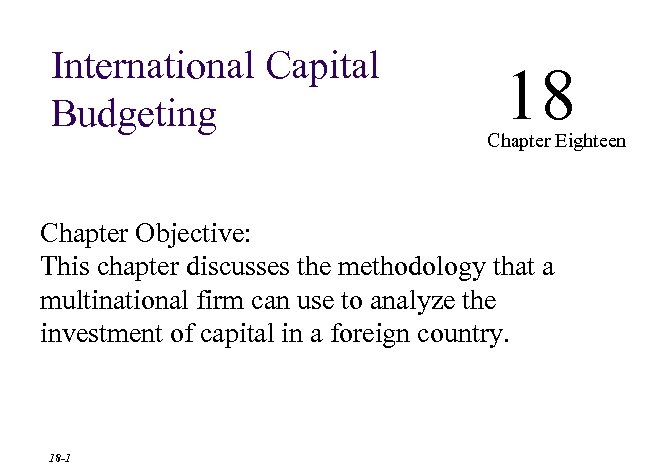International Capital Budgeting 18 Chapter Eighteen Chapter Objective: This chapter discusses the methodology that a multinational firm can use to analyze the Fifth investment of capital in a foreign country. Edition EUN / RESNICK 18 -1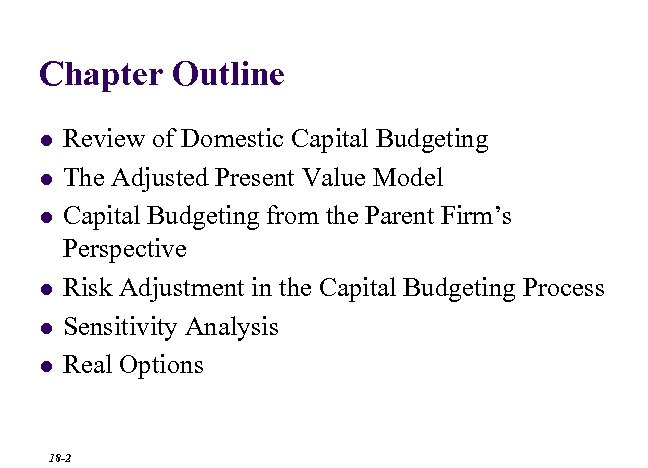Chapter Outline l l l Review of Domestic Capital Budgeting The Adjusted Present Value Model Capital Budgeting from the Parent Firm’s Perspective Risk Adjustment in the Capital Budgeting Process Sensitivity Analysis Real Options 18 -2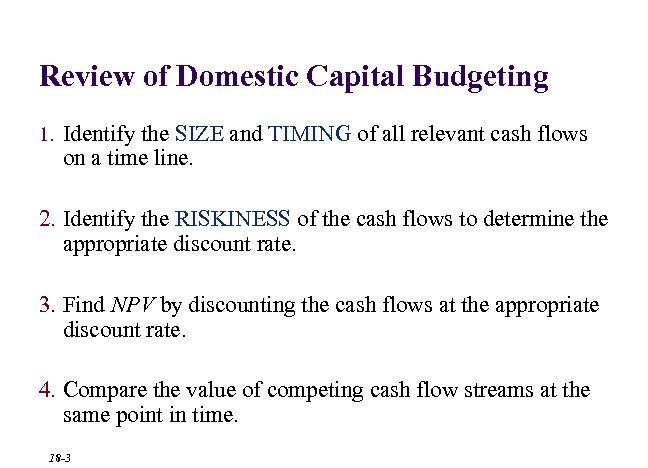Review of Domestic Capital Budgeting 1. Identify the SIZE and TIMING of all relevant cash flows on a time line. 2. Identify the RISKINESS of the cash flows to determine the appropriate discount rate. 3. Find NPV by discounting the cash flows at the appropriate discount rate. 4. Compare the value of competing cash flow streams at the same point in time. 18 -3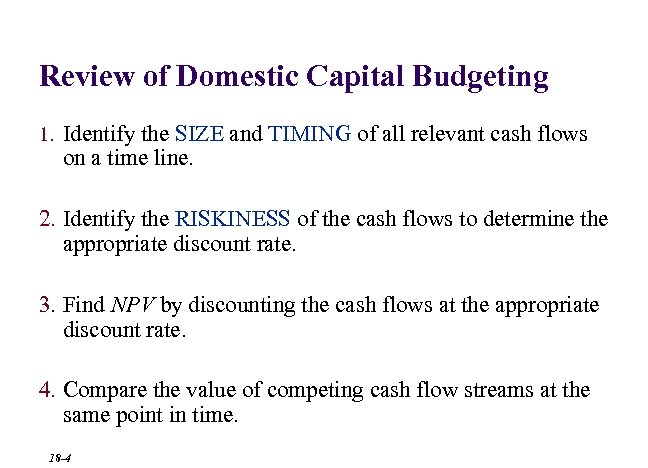Review of Domestic Capital Budgeting 1. Identify the SIZE and TIMING of all relevant cash flows on a time line. 2. Identify the RISKINESS of the cash flows to determine the appropriate discount rate. 3. Find NPV by discounting the cash flows at the appropriate discount rate. 4. Compare the value of competing cash flow streams at the same point in time. 18 -4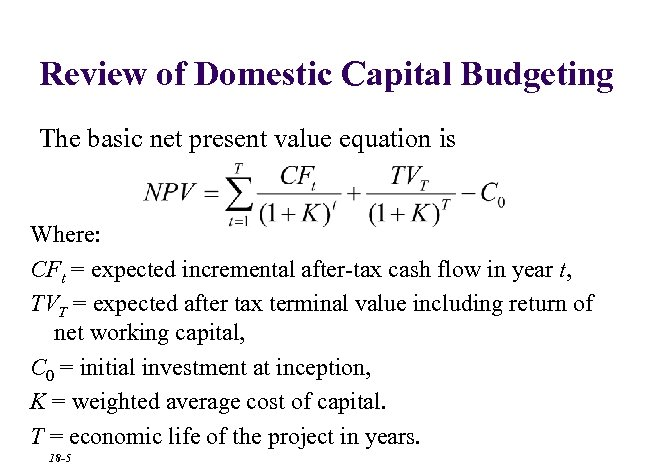Review of Domestic Capital Budgeting The basic net present value equation is Where: CFt = expected incremental after-tax cash flow in year t, TVT = expected after tax terminal value including return of net working capital, C 0 = initial investment at inception, K = weighted average cost of capital. T = economic life of the project in years. 18 -5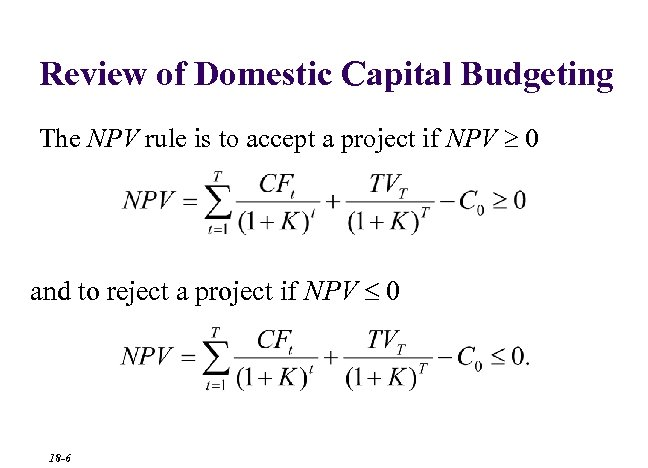Review of Domestic Capital Budgeting The NPV rule is to accept a project if NPV 0 and to reject a project if NPV 0 18 -6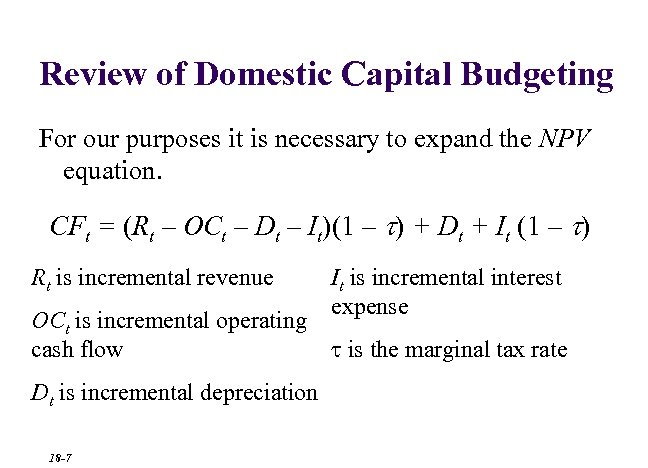Review of Domestic Capital Budgeting For our purposes it is necessary to expand the NPV equation. CFt = (Rt – OCt – Dt – It)(1 – t) + Dt + It (1 – t) Rt is incremental revenue OCt is incremental operating cash flow Dt is incremental depreciation 18 -7 It is incremental interest expense is the marginal tax rate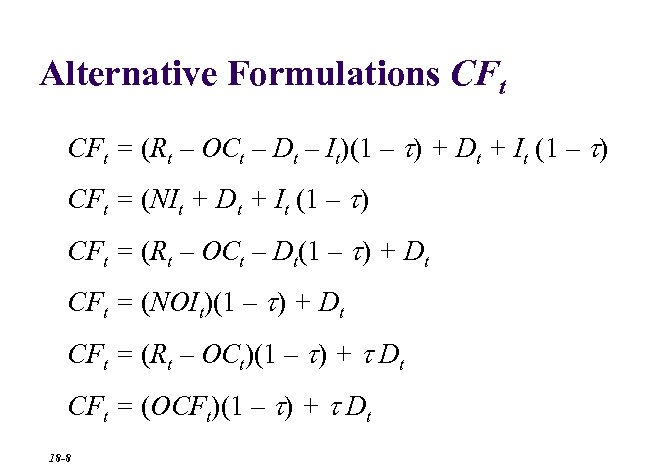Alternative Formulations CFt = (Rt – OCt – Dt – It)(1 – t) + Dt + It (1 – t) CFt = (NIt + Dt + It (1 – t) CFt = (Rt – OCt – Dt(1 – t) + Dt CFt = (NOIt)(1 – t) + Dt CFt = (Rt – OCt)(1 – t) + t Dt CFt = (OCFt)(1 – t) + t Dt 18 -8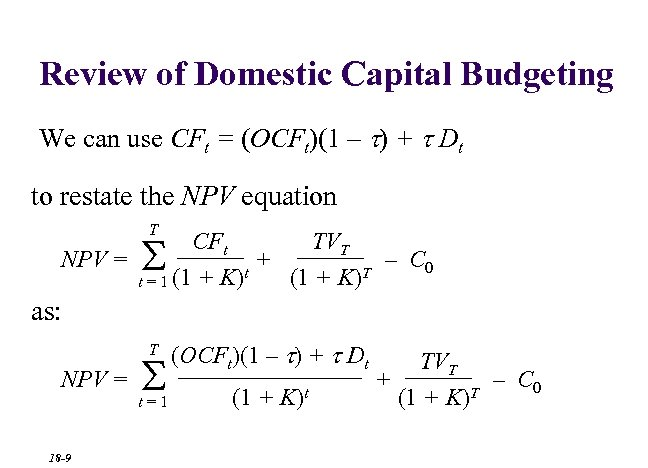Review of Domestic Capital Budgeting We can use CFt = (OCFt)(1 – t) + t Dt to restate the NPV equation T S CFt TVT NPV = + – C 0 t T (1 + K) t = 1 (1 + K) as: T t=1 NPV = 18 -9 (OCFt)(1 – t) + t Dt (1 + K)t S TVT + – C 0 T (1 + K)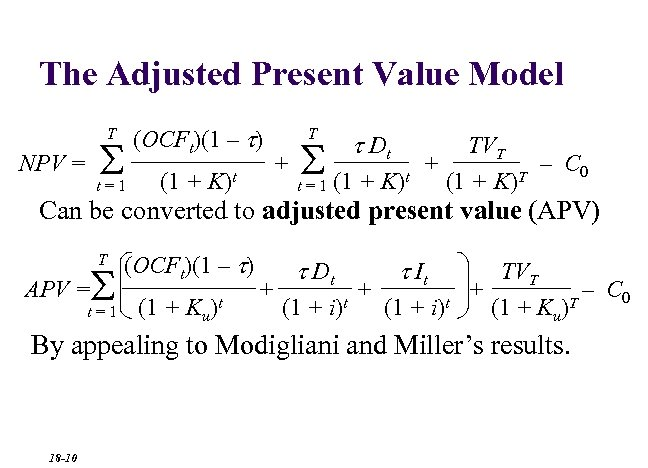The Adjusted Present Value Model T S NPV = t=1 (OCFt)(1 – t) (1 + K)t T t Dt S TVT + + – C 0 t T (1 + K) t = 1 (1 + K) Can be converted to adjusted present value (APV) T S APV = t=1 (OCFt)(1 – t) (1 + Ku)t + t Dt (1 + i)t + t It (1 + i)t TVT + – C 0 T (1 + Ku) By appealing to Modigliani and Miller’s results. 18 -10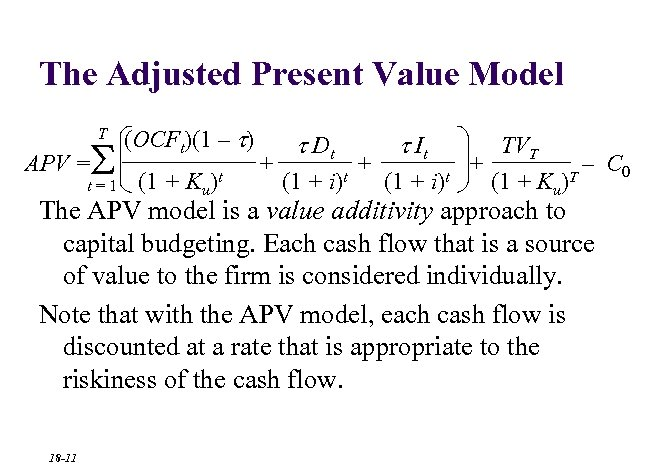The Adjusted Present Value Model T S APV = t=1 (OCFt)(1 – t) (1 + Ku)t + t Dt (1 + i)t + t It (1 + i)t TVT + – C 0 T (1 + Ku) The APV model is a value additivity approach to capital budgeting. Each cash flow that is a source of value to the firm is considered individually. Note that with the APV model, each cash flow is discounted at a rate that is appropriate to the riskiness of the cash flow. 18 -11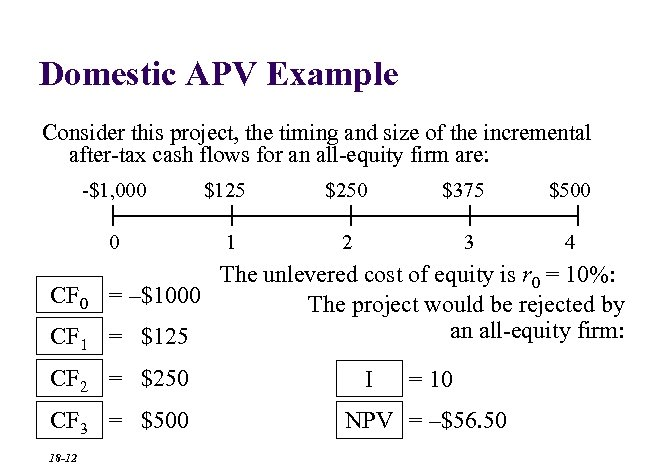Domestic APV Example Consider this project, the timing and size of the incremental after-tax cash flows for an all-equity firm are: -\$1, 000 \$125 \$250 \$375 \$500 0 1 2 3 4 CF 0 CF 1 The unlevered cost of equity is r 0 = 10%: = –\$1000 The project would be rejected by an all-equity firm: = \$125 CF 2 = \$250 CF 3 = \$500 18 -12 I = 10 NPV = –\$56. 50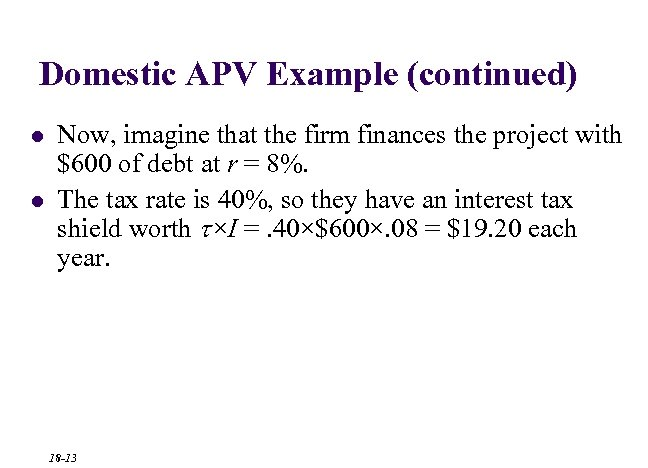Domestic APV Example (continued) l l Now, imagine that the firm finances the project with \$600 of debt at r = 8%. The tax rate is 40%, so they have an interest tax shield worth t×I =. 40×\$600×. 08 = \$19. 20 each year. 18 -13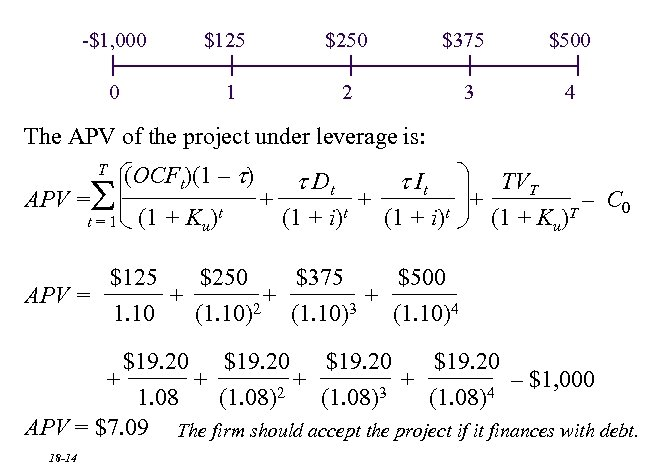-\$1, 000 \$125 \$250 \$375 \$500 0 1 2 3 4 The APV of the project under leverage is: T S APV = t=1 (OCFt)(1 – t) (1 + Ku)t + t Dt (1 + i)t + t It (1 + i)t TVT + – C 0 T (1 + Ku) \$125 \$250 \$375 \$500 APV = + + + 2 3 1. 10 (1. 10)4 \$19. 20 + + – \$1, 000 2 3 4 1. 08 (1. 08) APV = \$7. 09 The firm should accept the project if it finances with debt. 18 -14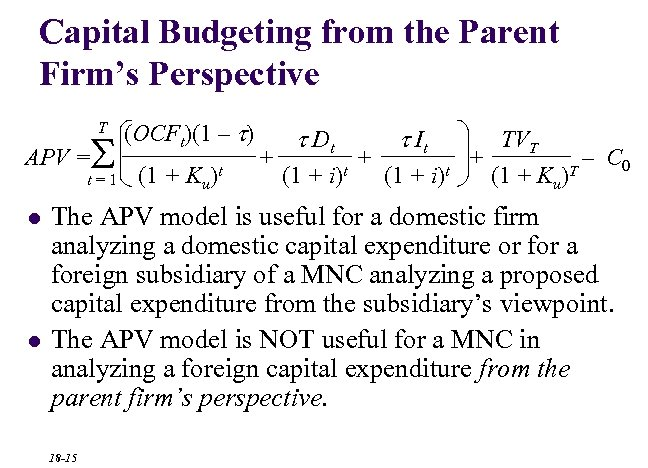Capital Budgeting from the Parent Firm’s Perspective T S APV = t=1 l l (OCFt)(1 – t) (1 + Ku)t + t Dt (1 + i)t + t It (1 + i)t TVT + – C 0 T (1 + Ku) The APV model is useful for a domestic firm analyzing a domestic capital expenditure or for a foreign subsidiary of a MNC analyzing a proposed capital expenditure from the subsidiary’s viewpoint. The APV model is NOT useful for a MNC in analyzing a foreign capital expenditure from the parent firm’s perspective. 18 -15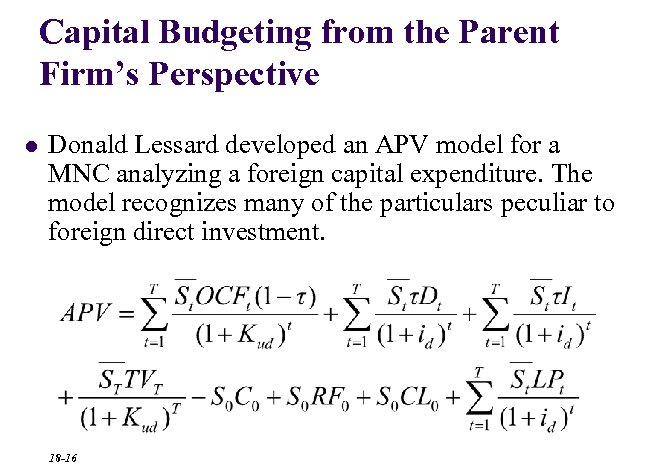Capital Budgeting from the Parent Firm’s Perspective l Donald Lessard developed an APV model for a MNC analyzing a foreign capital expenditure. The model recognizes many of the particulars peculiar to foreign direct investment. 18 -16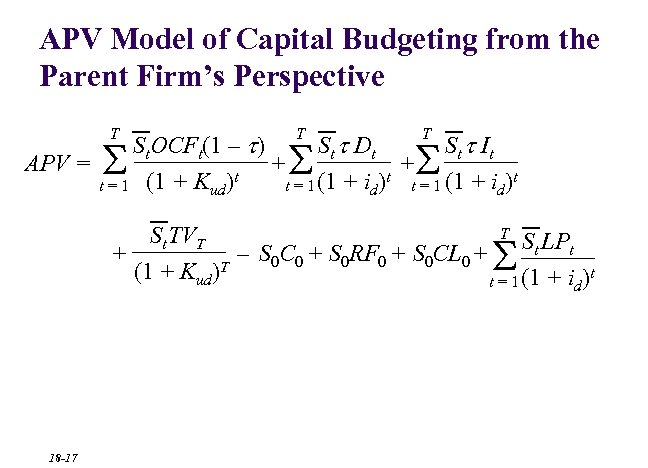APV Model of Capital Budgeting from the Parent Firm’s Perspective T T T St. OCFt(1 – t) St t Dt St t It APV = + + t t = 1 (1 + Kud) t = 1 (1 + id) S S S T St TVT + – S 0 C 0 + S 0 RF 0 + S 0 CL 0 + St LPt t (1 + Kud)T t = 1 (1 + id) S 18 -17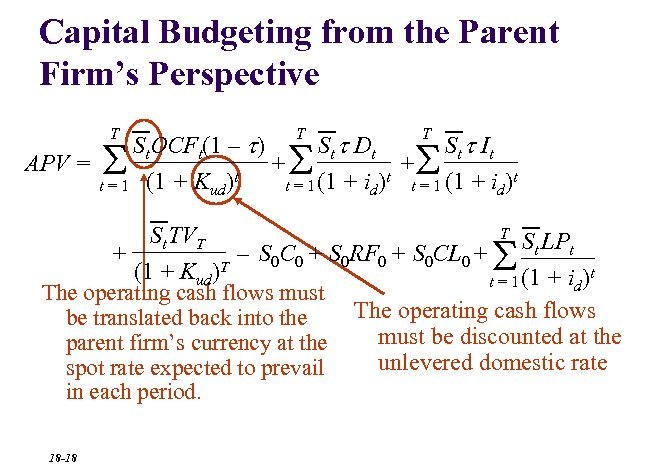Capital Budgeting from the Parent Firm’s Perspective T T T St. OCFt(1 – t) St t Dt St t It APV = + + t t = 1 (1 + Kud) t = 1 (1 + id) S S S T St TVT + – S 0 C 0 + S 0 RF 0 + S 0 CL 0 + St LPt t (1 + Kud)T t = 1 (1 + id) The operating cash flows must The operating cash flows be translated back into the must be discounted at the parent firm’s currency at the unlevered domestic rate spot rate expected to prevail in each period. S 18 -18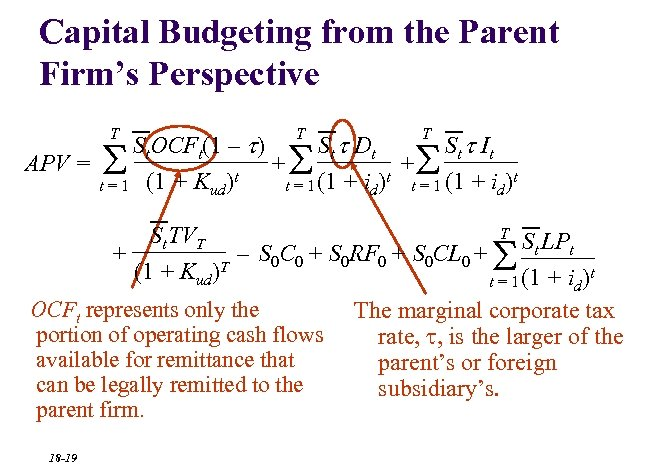Capital Budgeting from the Parent Firm’s Perspective T T T St. OCFt(1 – t) St t Dt St t It APV = + + t t = 1 (1 + Kud) t = 1 (1 + id) S S S T St TVT + – S 0 C 0 + S 0 RF 0 + S 0 CL 0 + St LPt t (1 + Kud)T t = 1 (1 + id) S OCFt represents only the portion of operating cash flows available for remittance that can be legally remitted to the parent firm. 18 -19 The marginal corporate tax rate, , is the larger of the parent’s or foreign subsidiary’s.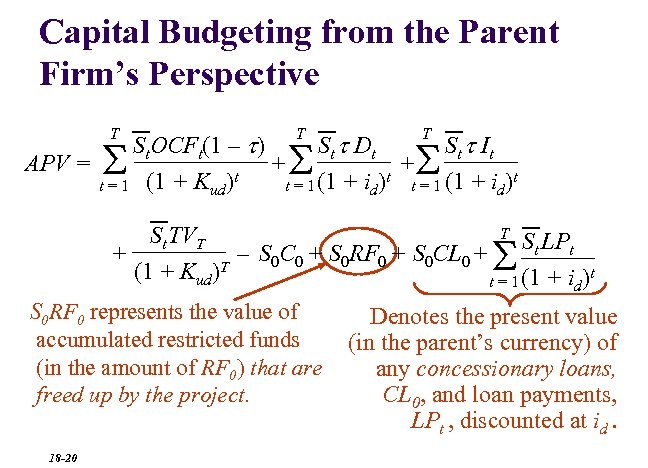Capital Budgeting from the Parent Firm’s Perspective T T T St. OCFt(1 – t) St t Dt St t It APV = + + t t = 1 (1 + Kud) t = 1 (1 + id) S S S T St TVT + – S 0 C 0 + S 0 RF 0 + S 0 CL 0 + St LPt t (1 + Kud)T t = 1 (1 + id) S S 0 RF 0 represents the value of accumulated restricted funds (in the amount of RF 0) that are freed up by the project. 18 -20 Denotes the present value (in the parent’s currency) of any concessionary loans, CL 0, and loan payments, LPt , discounted at id.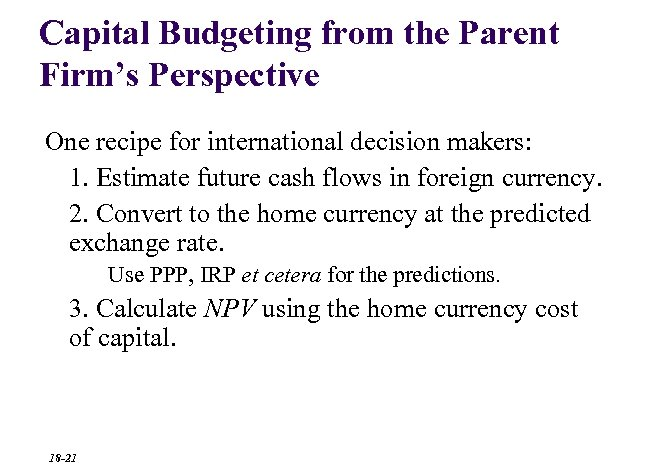Capital Budgeting from the Parent Firm’s Perspective One recipe for international decision makers: 1. Estimate future cash flows in foreign currency. 2. Convert to the home currency at the predicted exchange rate. Use PPP, IRP et cetera for the predictions. 3. Calculate NPV using the home currency cost of capital. 18 -21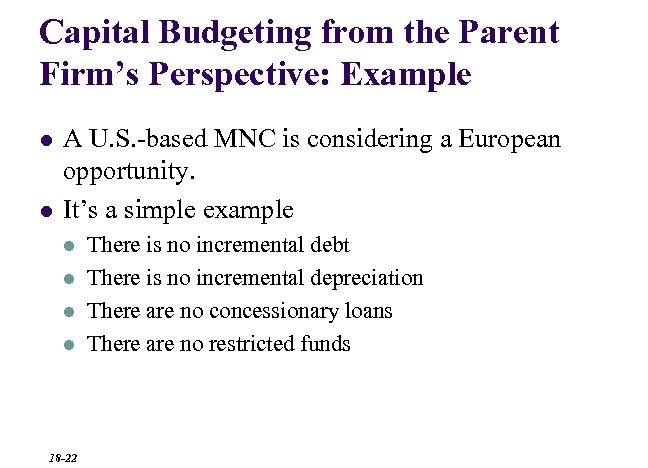Capital Budgeting from the Parent Firm’s Perspective: Example l l A U. S. -based MNC is considering a European opportunity. It’s a simple example l l 18 -22 There is no incremental debt There is no incremental depreciation There are no concessionary loans There are no restricted funds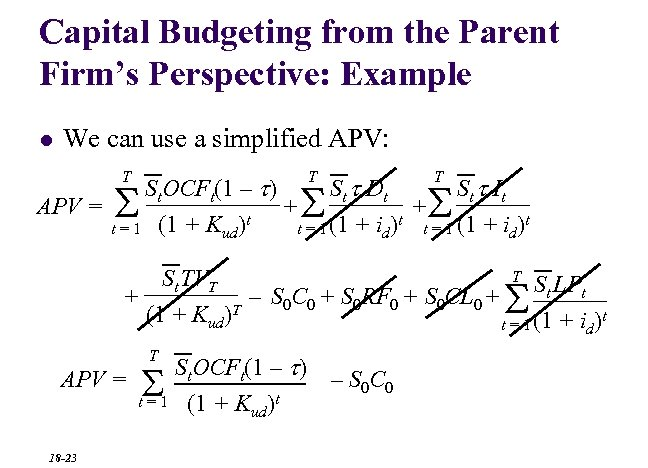Capital Budgeting from the Parent Firm’s Perspective: Example l We can use a simplified APV: T T T St. OCFt(1 – t) St t Dt St t It APV = + + t t = 1 (1 + Kud) t = 1 (1 + id) S S S T St TVT + – S 0 C 0 + S 0 RF 0 + S 0 CL 0 + St LPt t (1 + Kud)T t = 1 (1 + id) S T APV = S t=1 18 -23 St. OCFt(1 – t) (1 + Kud)t – S 0 C 0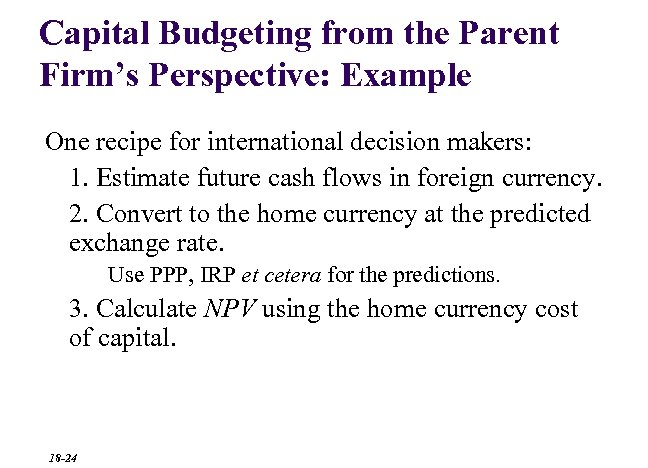Capital Budgeting from the Parent Firm’s Perspective: Example One recipe for international decision makers: 1. Estimate future cash flows in foreign currency. 2. Convert to the home currency at the predicted exchange rate. Use PPP, IRP et cetera for the predictions. 3. Calculate NPV using the home currency cost of capital. 18 -24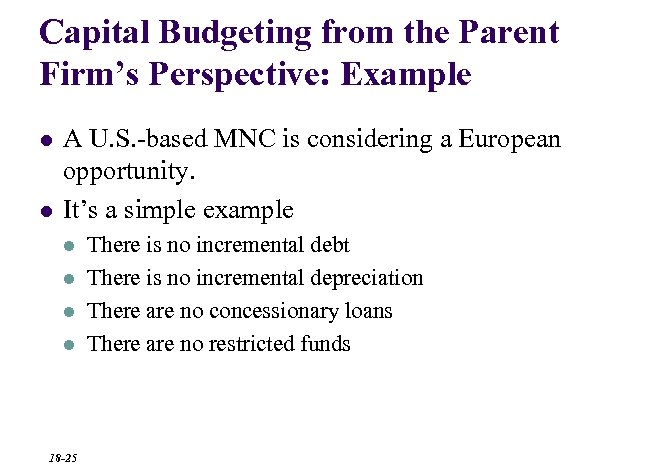Capital Budgeting from the Parent Firm’s Perspective: Example l l A U. S. -based MNC is considering a European opportunity. It’s a simple example l l 18 -25 There is no incremental debt There is no incremental depreciation There are no concessionary loans There are no restricted funds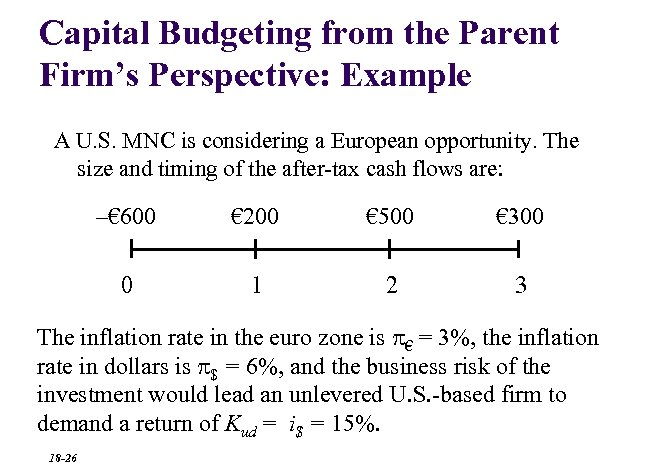Capital Budgeting from the Parent Firm’s Perspective: Example A U. S. MNC is considering a European opportunity. The size and timing of the after-tax cash flows are: –€ 600 € 200 € 500 € 300 0 1 2 3 The inflation rate in the euro zone is € = 3%, the inflation rate in dollars is \$ = 6%, and the business risk of the investment would lead an unlevered U. S. -based firm to demand a return of Kud = i\$ = 15%. 18 -26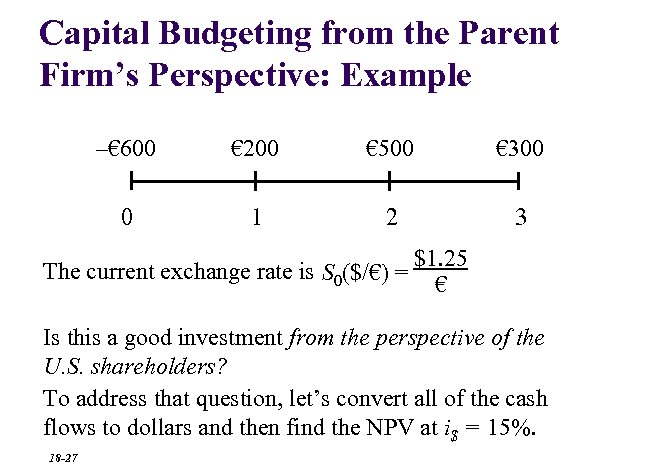Capital Budgeting from the Parent Firm’s Perspective: Example –€ 600 € 200 € 500 € 300 0 1 2 3 The current exchange rate is S 0(\$/€) = \$1. 25 € Is this a good investment from the perspective of the U. S. shareholders? To address that question, let’s convert all of the cash flows to dollars and then find the NPV at i\$ = 15%. 18 -27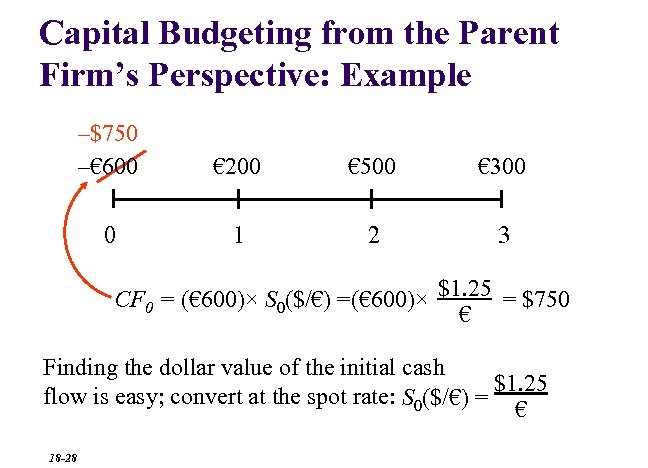Capital Budgeting from the Parent Firm’s Perspective: Example –\$750 –€ 600 € 200 € 500 € 300 0 1 2 3 CF 0 = (€ 600)× S 0(\$/€) =(€ 600)× \$1. 25 = \$750 € Finding the dollar value of the initial cash \$1. 25 flow is easy; convert at the spot rate: S 0(\$/€) = € 18 -28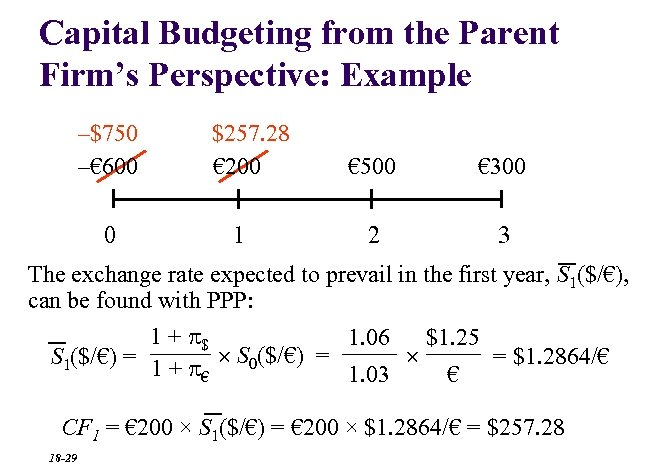Capital Budgeting from the Parent Firm’s Perspective: Example –\$750 –€ 600 0 \$257. 28 € 200 1 € 500 € 300 2 3 The exchange rate expected to prevail in the first year, S 1(\$/€), can be found with PPP: 1 + \$ 1. 06 \$1. 25 S 1(\$/€) = 1 + S 0(\$/€) = = \$1. 2864/€ € 1. 03 € CF 1 = € 200 × S 1(\$/€) = € 200 × \$1. 2864/€ = \$257. 28 18 -29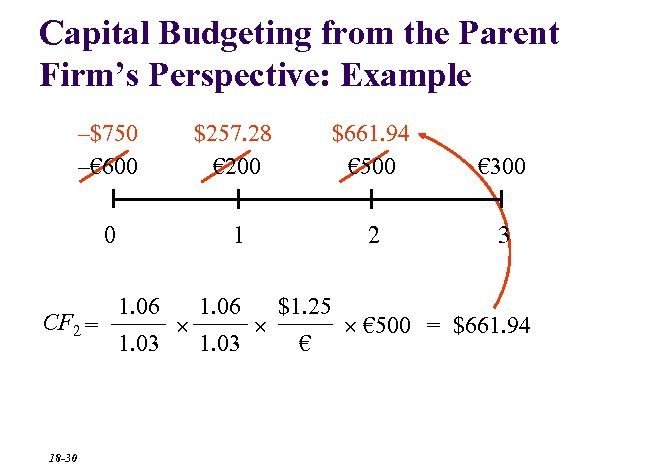Capital Budgeting from the Parent Firm’s Perspective: Example –\$750 –€ 600 \$257. 28 € 200 \$661. 94 € 500 € 300 0 1 2 3 CF 2 = 18 -30 1. 06 1. 03 \$1. 25 € € 500 = \$661. 94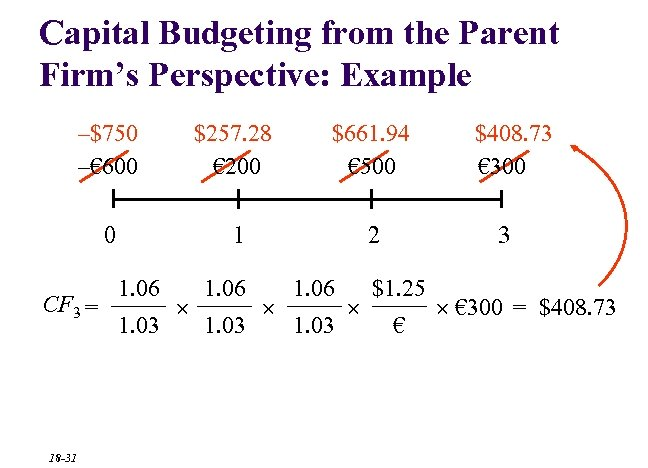Capital Budgeting from the Parent Firm’s Perspective: Example –\$750 –€ 600 \$257. 28 € 200 \$661. 94 € 500 0 1 2 CF 3 = 18 -31 1. 06 1. 03 \$408. 73 € 300 3 \$1. 25 € € 300 = \$408. 73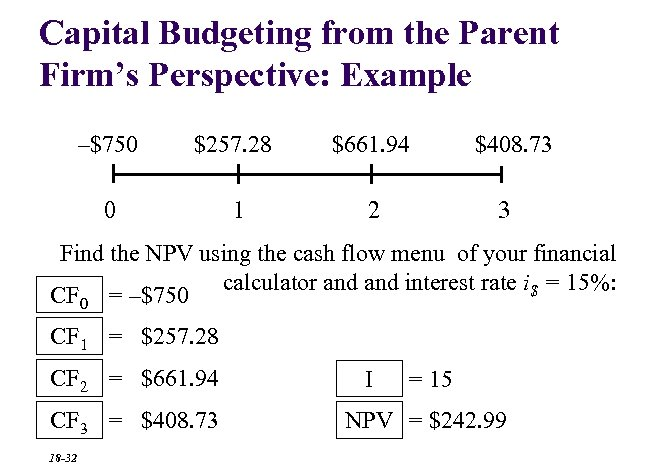Capital Budgeting from the Parent Firm’s Perspective: Example –\$750 \$257. 28 \$661. 94 0 1 2 \$408. 73 3 Find the NPV using the cash flow menu of your financial calculator and interest rate i\$ = 15%: CF 0 = –\$750 CF 1 = \$257. 28 CF 2 = \$661. 94 CF 3 = \$408. 73 18 -32 I = 15 NPV = \$242. 99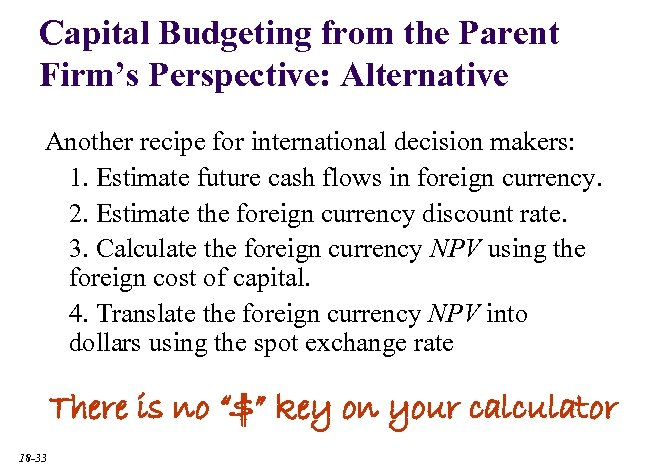Capital Budgeting from the Parent Firm’s Perspective: Alternative Another recipe for international decision makers: 1. Estimate future cash flows in foreign currency. 2. Estimate the foreign currency discount rate. 3. Calculate the foreign currency NPV using the foreign cost of capital. 4. Translate the foreign currency NPV into dollars using the spot exchange rate There is no “\$” key on your calculator 18 -33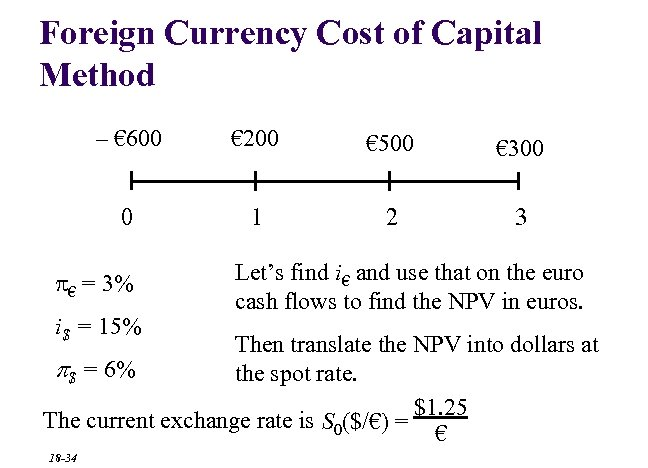Foreign Currency Cost of Capital Method – € 600 € 200 € 500 € 300 0 1 2 3 € = 3% i\$ = 15% Let’s find i€ and use that on the euro cash flows to find the NPV in euros. Then translate the NPV into dollars at p\$ = 6% the spot rate. \$1. 25 The current exchange rate is S 0(\$/€) = € 18 -34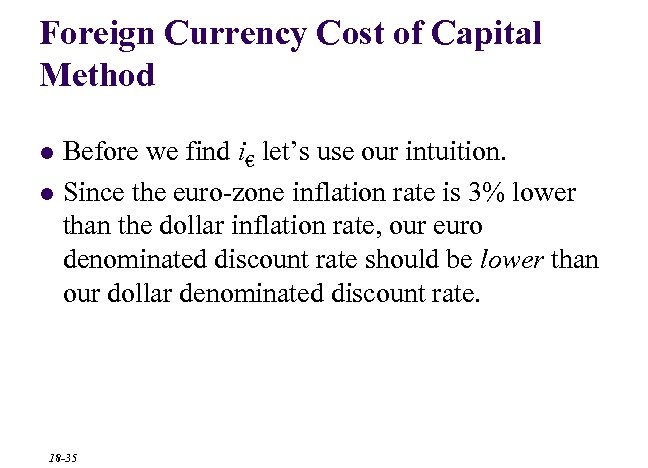Foreign Currency Cost of Capital Method l l Before we find i€ let’s use our intuition. Since the euro-zone inflation rate is 3% lower than the dollar inflation rate, our euro denominated discount rate should be lower than our dollar denominated discount rate. 18 -35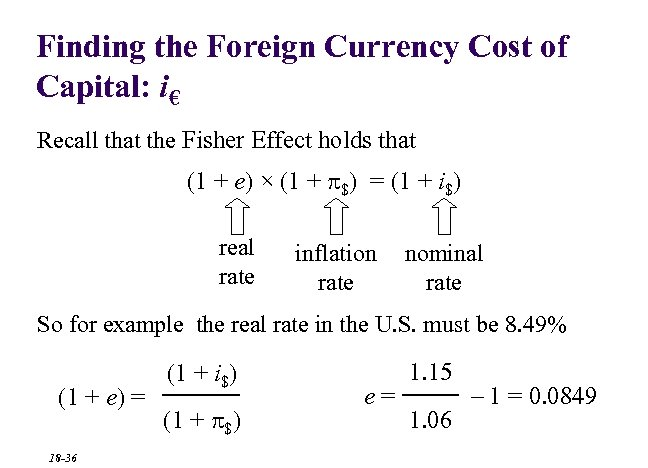Finding the Foreign Currency Cost of Capital: i€ Recall that the Fisher Effect holds that (1 + e) × (1 + \$) = (1 + i\$) real rate inflation rate nominal rate So for example the real rate in the U. S. must be 8. 49% (1 + e) = 18 -36 (1 + i\$) (1 + \$) e= 1. 15 1. 06 – 1 = 0. 0849Finding the Foreign Currency Cost of Capital: i€ If Fisher Effect holds here and abroad then (1 + e\$) = (1 + i\$) (1 + \$) and (1 + e€) = (1 + i€) (1 + €) If the real rates are the same in dollars and euros (e€ = e\$) we have a very useful parity condition: (1 + i\$) (1 + \$) 18 -37 = (1 + i€) (1 + €)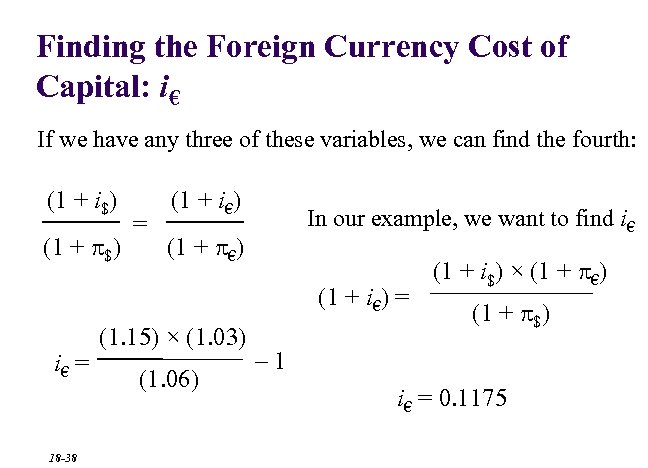Finding the Foreign Currency Cost of Capital: i€ If we have any three of these variables, we can find the fourth: (1 + i\$) (1 + \$) = (1 + i€) In our example, we want to find i€ (1 + €) (1 + i€) = i€ = 18 -38 (1. 15) × (1. 03) (1. 06) (1 + i\$) × (1 + €) (1 + \$) – 1 i€ = 0. 1175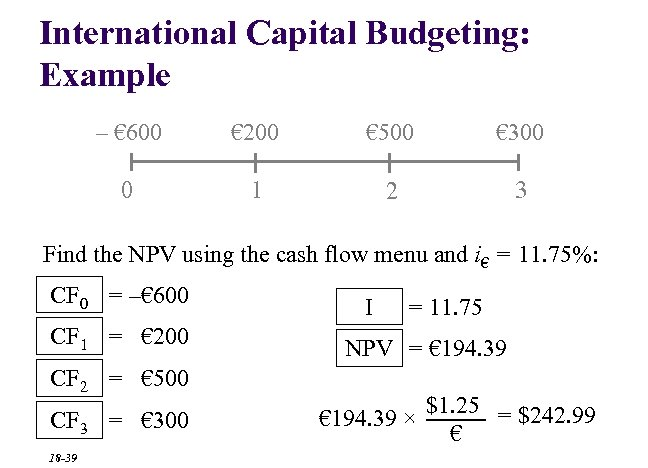International Capital Budgeting: Example – € 600 € 200 € 500 € 300 0 1 2 3 Find the NPV using the cash flow menu and i€ = 11. 75%: CF 0 = –€ 600 CF 1 = € 200 I = 11. 75 NPV = € 194. 39 CF 2 = € 500 CF 3 = € 300 18 -39 € 194. 39 × \$1. 25 = \$242. 99 €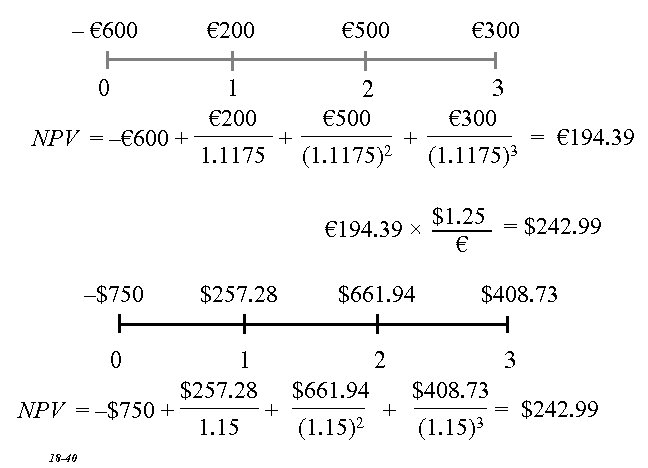– € 600 € 200 € 500 € 300 0 1 3 2 € 200 € 500 € 300 = € 194. 39 + + NPV = –€ 600 + 2 3 1. 1175 (1. 1175) € 194. 39 × –\$750 0 \$257. 28 \$1. 25 = \$242. 99 € \$661. 94 \$408. 73 1 3 2 \$257. 28 \$661. 94 \$408. 73 = \$242. 99 + + NPV = –\$750 + 2 3 1. 15 (1. 15) 18 -40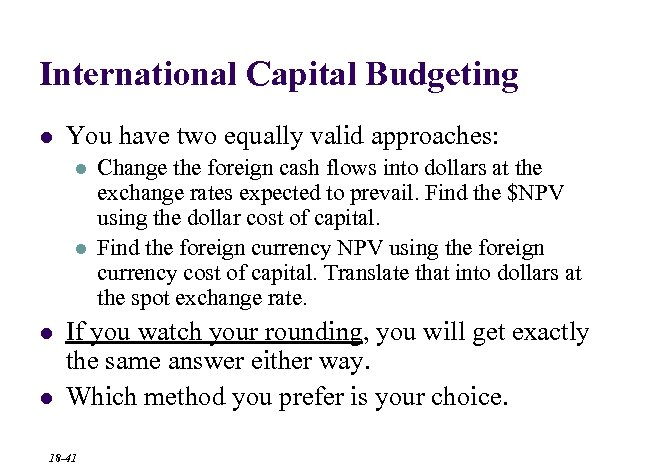International Capital Budgeting l You have two equally valid approaches: l l Change the foreign cash flows into dollars at the exchange rates expected to prevail. Find the \$NPV using the dollar cost of capital. Find the foreign currency NPV using the foreign currency cost of capital. Translate that into dollars at the spot exchange rate. If you watch your rounding, you will get exactly the same answer either way. Which method you prefer is your choice. 18 -41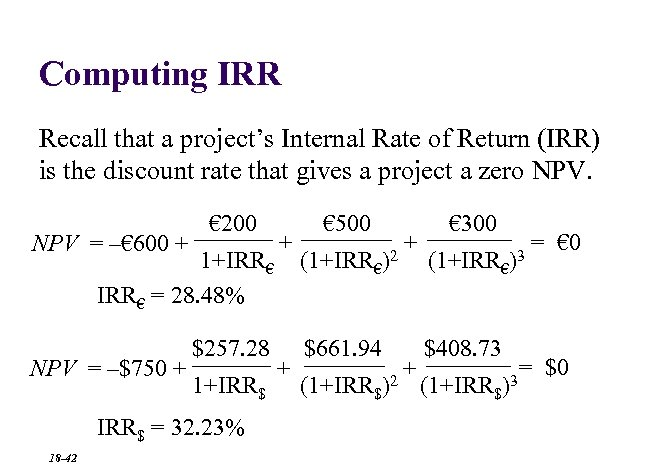Computing IRR Recall that a project’s Internal Rate of Return (IRR) is the discount rate that gives a project a zero NPV = –€ 600 + € 200 1+IRR€ = 28. 48% NPV = –\$750 + \$257. 28 1+IRR\$ = 32. 23% 18 -42 + + € 500 (1+IRR€)2 \$661. 94 (1+IRR\$)2 + + € 300 (1+IRR€)3 \$408. 73 (1+IRR\$)3 = € 0 = \$0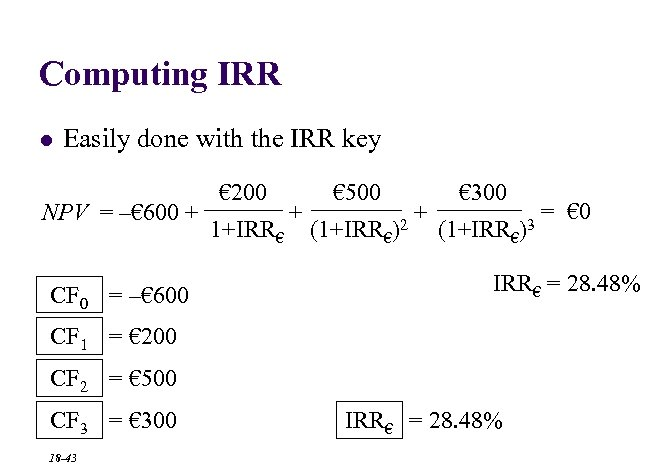Computing IRR l Easily done with the IRR key NPV = –€ 600 + CF 0 = –€ 600 € 200 1+IRR€ + € 500 (1+IRR€)2 + € 300 (1+IRR€)3 IRR€ = 28. 48% CF 1 = € 200 CF 2 = € 500 CF 3 = € 300 18 -43 = € 0 IRR€ = 28. 48%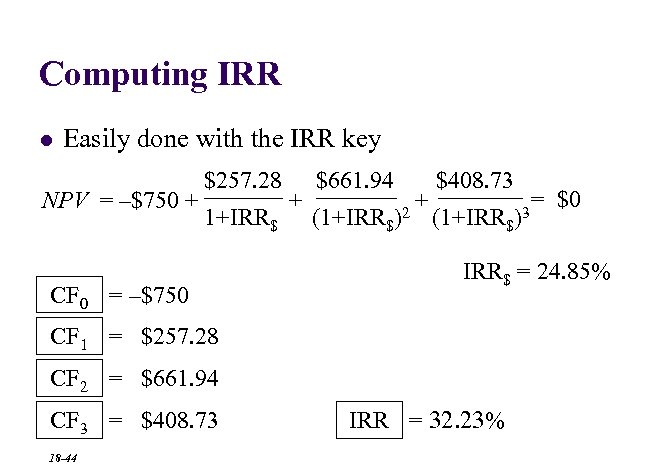Computing IRR l Easily done with the IRR key NPV = –\$750 + \$257. 28 1+IRR\$ CF 0 = –\$750 + \$661. 94 (1+IRR\$)2 + \$408. 73 (1+IRR\$)3 IRR\$ = 24. 85% CF 1 = \$257. 28 CF 2 = \$661. 94 CF 3 = \$408. 73 18 -44 = \$0 IRR = 32. 23%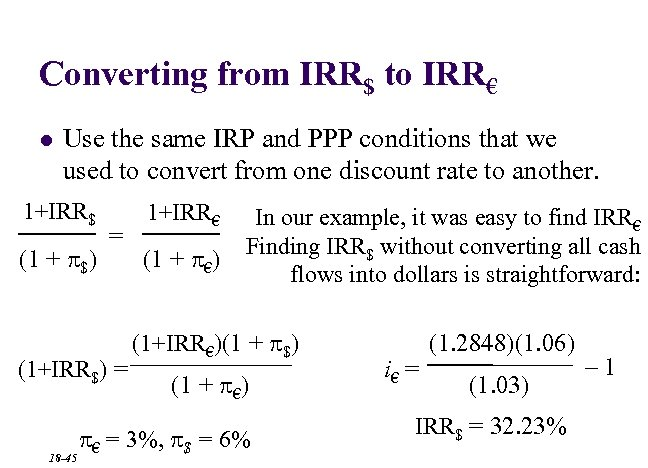Converting from IRR\$ to IRR€ l Use the same IRP and PPP conditions that we used to convert from one discount rate to another. 1+IRR\$ (1 + \$) = (1+IRR\$) = 18 -45 1+IRR€ (1 + €) In our example, it was easy to find IRR€ Finding IRR\$ without converting all cash flows into dollars is straightforward: (1+IRR€)(1 + \$) (1 + €) € = 3%, \$ = 6% i€ = (1. 2848)(1. 06) (1. 03) IRR\$ = 32. 23% – 1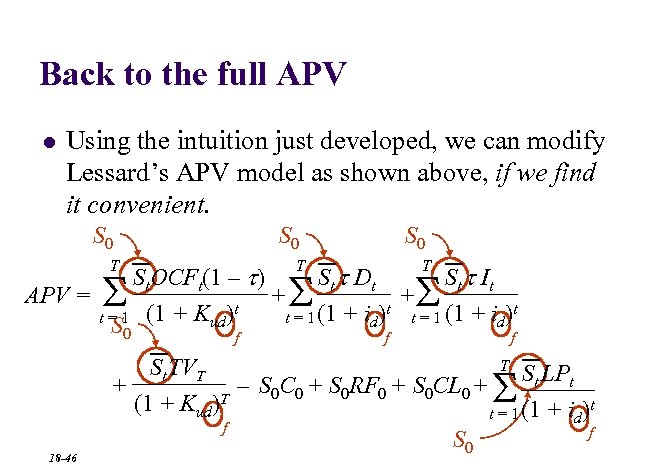Back to the full APV l Using the intuition just developed, we can modify Lessard’s APV model as shown above, if we find it convenient. S 0 T T St. OCFt(1 – t) St t Dt St t It APV = + + t t = 1 (1 + Kud) t = 1 (1 + id) S S 0 S f f T St TVT + – S 0 C 0 + S 0 RF 0 + S 0 CL 0 + St LPt t (1 + Kud)T t = 1 (1 + id) f f S 0 S 18 -46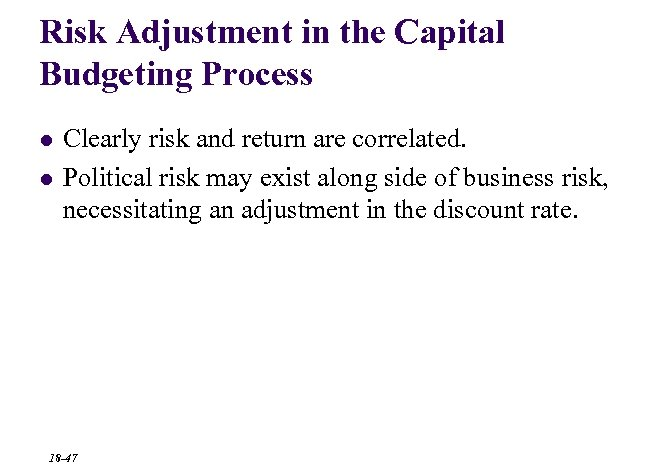Risk Adjustment in the Capital Budgeting Process l l Clearly risk and return are correlated. Political risk may exist along side of business risk, necessitating an adjustment in the discount rate. 18 -47Sensitivity Analysis l l In sensitivity analysis, different estimates are used for expected inflation rates, cost and pricing estimates, and other inputs to give the manager a more complete picture of the planned capital investment. Lends itself to computer simulation. 18 -48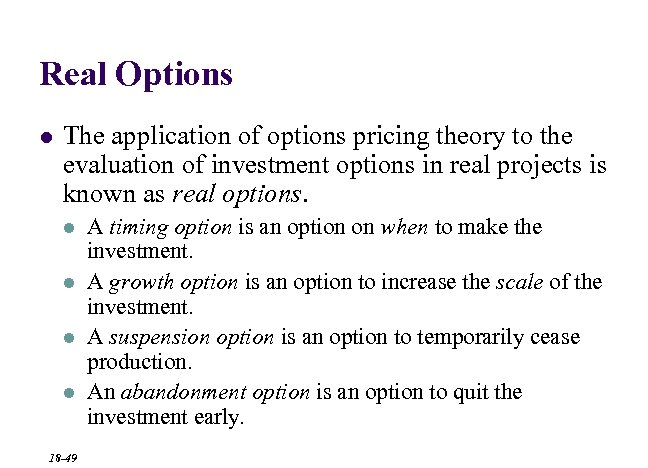Real Options l The application of options pricing theory to the evaluation of investment options in real projects is known as real options. l l 18 -49 A timing option is an option on when to make the investment. A growth option is an option to increase the scale of the investment. A suspension option is an option to temporarily cease production. An abandonment option is an option to quit the investment early.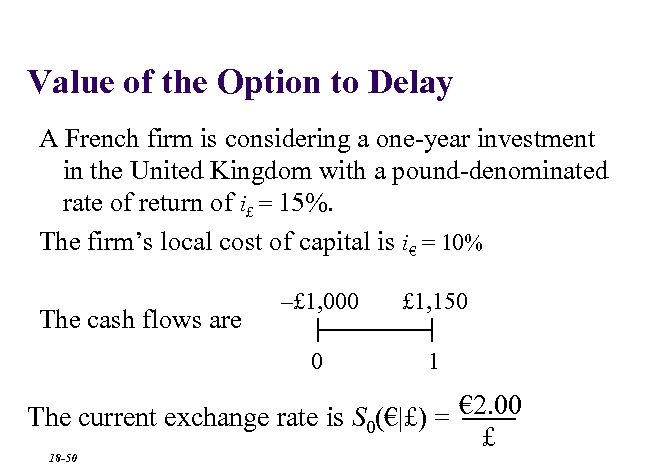Value of the Option to Delay A French firm is considering a one-year investment in the United Kingdom with a pound-denominated rate of return of i£ = 15%. The firm’s local cost of capital is i€ = 10% £ 1, 150 0 The cash flows are –£ 1, 000 1 The current exchange rate is S 0(€|£) = € 2. 00 £ 18 -50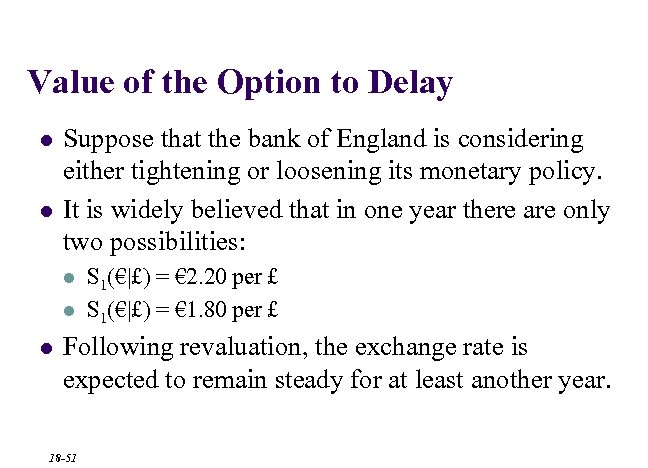Value of the Option to Delay l l Suppose that the bank of England is considering either tightening or loosening its monetary policy. It is widely believed that in one year there are only two possibilities: l l l S 1(€|£) = € 2. 20 per £ S 1(€|£) = € 1. 80 per £ Following revaluation, the exchange rate is expected to remain steady for at least another year. 18 -51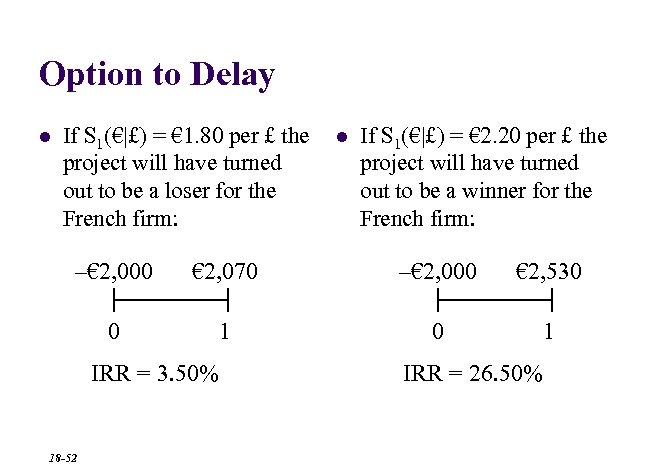Option to Delay l If S 1(€|£) = € 1. 80 per £ the project will have turned out to be a loser for the French firm: l If S 1(€|£) = € 2. 20 per £ the project will have turned out to be a winner for the French firm: –€ 2, 000 € 2, 070 –€ 2, 000 € 2, 530 0 1 IRR = 3. 50% 18 -52 IRR = 26. 50%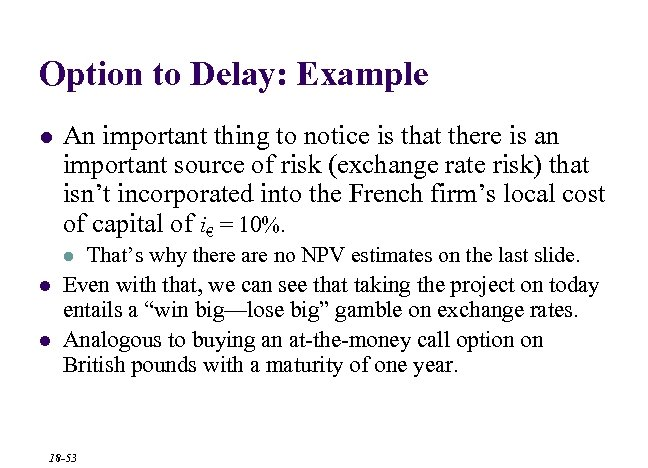Option to Delay: Example l An important thing to notice is that there is an important source of risk (exchange rate risk) that isn’t incorporated into the French firm’s local cost of capital of i€ = 10%. That’s why there are no NPV estimates on the last slide. Even with that, we can see that taking the project on today entails a “win big—lose big” gamble on exchange rates. Analogous to buying an at-the-money call option on British pounds with a maturity of one year. l l l 18 -53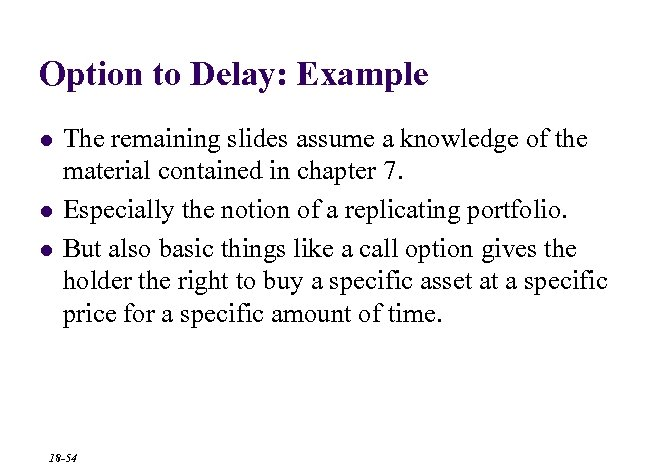Option to Delay: Example l l l The remaining slides assume a knowledge of the material contained in chapter 7. Especially the notion of a replicating portfolio. But also basic things like a call option gives the holder the right to buy a specific asset at a specific price for a specific amount of time. 18 -54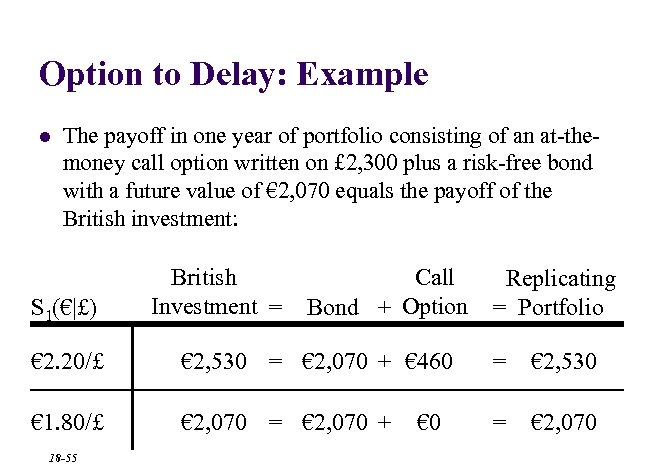Option to Delay: Example l The payoff in one year of portfolio consisting of an at-themoney call option written on £ 2, 300 plus a risk-free bond with a future value of € 2, 070 equals the payoff of the British investment: S 1(€|£) British Investment = Call Bond + Option Replicating = Portfolio € 2. 20/£ € 2, 530 = € 2, 070 + € 460 = € 2, 530 € 1. 80/£ € 2, 070 = € 2, 070 + = € 2, 070 18 -55 € 0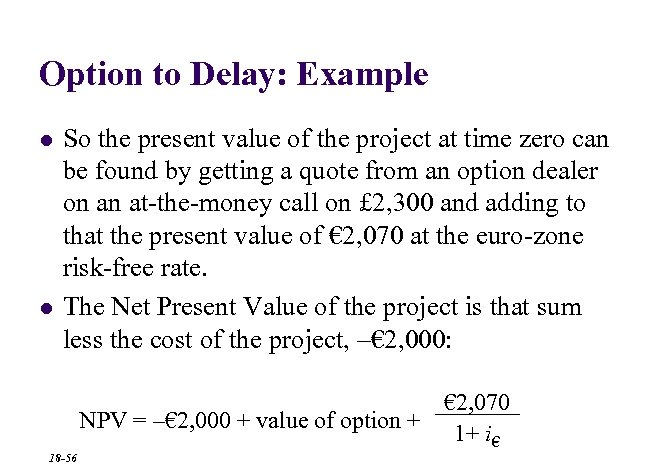Option to Delay: Example l l So the present value of the project at time zero can be found by getting a quote from an option dealer on an at-the-money call on £ 2, 300 and adding to that the present value of € 2, 070 at the euro-zone risk-free rate. The Net Present Value of the project is that sum less the cost of the project, –€ 2, 000: € 2, 070 NPV = –€ 2, 000 + value of option + 1+ i€ 18 -56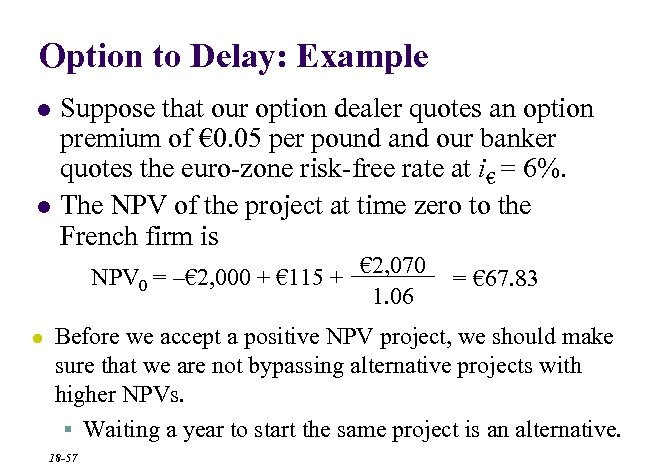Option to Delay: Example l l Suppose that our option dealer quotes an option premium of € 0. 05 per pound and our banker quotes the euro-zone risk-free rate at i€ = 6%. The NPV of the project at time zero to the French firm is NPV 0 = –€ 2, 000 + € 115 + € 2, 070 1. 06 l = € 67. 83 Before we accept a positive NPV project, we should make sure that we are not bypassing alternative projects with higher NPVs. § Waiting a year to start the same project is an alternative. 18 -57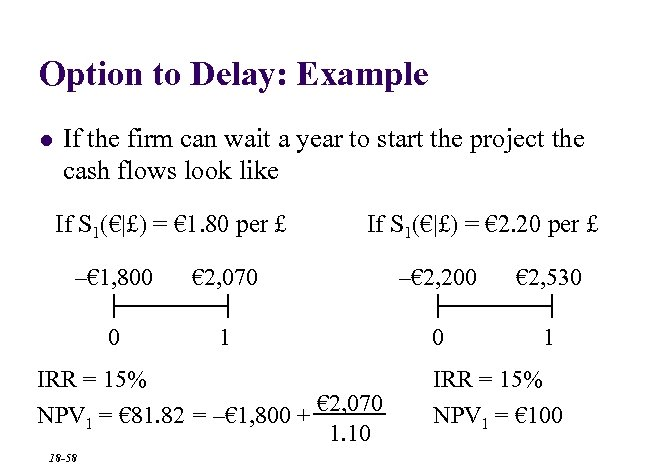Option to Delay: Example l If the firm can wait a year to start the project the cash flows look like If S 1(€|£) = € 1. 80 per £ If S 1(€|£) = € 2. 20 per £ –€ 1, 800 € 2, 070 –€ 2, 200 € 2, 530 0 1 IRR = 15% € 2, 070 NPV 1 = € 81. 82 = –€ 1, 800 + 1. 10 18 -58 IRR = 15% NPV 1 = € 100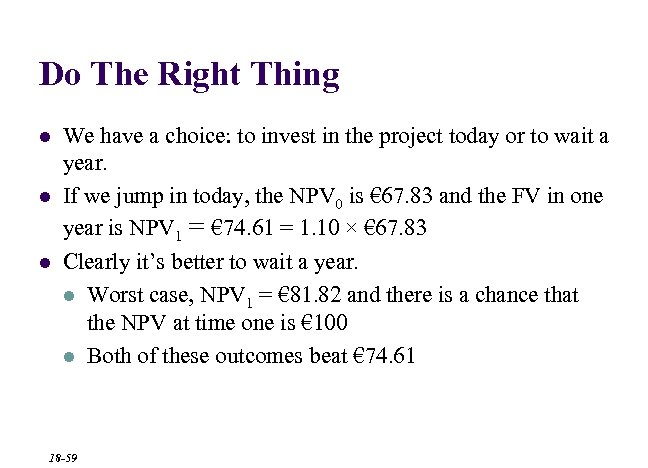Do The Right Thing l l l We have a choice: to invest in the project today or to wait a year. If we jump in today, the NPV 0 is € 67. 83 and the FV in one year is NPV 1 = € 74. 61 = 1. 10 × € 67. 83 Clearly it’s better to wait a year. l Worst case, NPV 1 = € 81. 82 and there is a chance that the NPV at time one is € 100 l Both of these outcomes beat € 74. 61 18 -59End Chapter Eighteen 18 -60